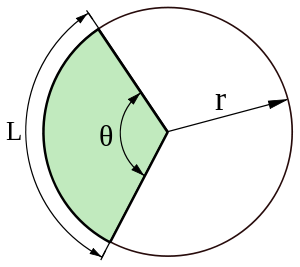GeeksforGeeks App
Open AppBrowser
Continue

# Area of a Circular Sector

A circular sector or circle sector is the portion of a disk enclosed by two radii and an arc, where the smaller area is known as the minor sector and the larger being the major sector. Let’s look at this figure and try to figure out the sector:source: Wikipedia ( https://goo.gl/mWijn2 )

In this figure the green shaded part is a sector, “r” is the Radius and “theta” is the angle as shown. Here, we can say that the shaded portion is the minor sector and the other portion is the major sector. “L” is the Arc of the Sector. For more, visit Sector
Now let’s see the formula using which the sector of a circle can be calculated.The area of the sector is similar to the calculation of the area of the circle, just multiply the area of the circle with the angle of the sector.
Examples:

```Input:
angle = 60
Explanation:
Sector = ( pi * 9*9 ) * ( 60 / 360 )
Output: 42.42857142857142

Input:
angle = 145
Explanation:
Sector = ( pi * 20*20 ) * ( 145 / 360 )
Output: 506.3492063492063```

## C++

 `// C++ program to find Area of a Sector``#include ``using` `namespace` `std;`` ` `void` `SectorArea(``double` `radius,``double` `angle)``{``    ``if``(angle >= 360)``        ``cout<<``"Angle not possible"``;``     ` `    ``// Calculating area of the sector``    ``else``    ``{``        ``double` `sector = ((22 * radius * radius) / 7) ``                       ``* (angle / 360);``        ``cout<

## Java

 `// Java program to find Area of a Sector`` ` `class` `GFG ``{``    ``static` `void` `SectorArea(``double` `radius,``double` `angle)``    ``{``        ``if``(angle >= ``360``)``            ``System.out.println(``"Angle not possible"``);``         ` `        ``// Calculating area of the sector``        ``else``        ``{``            ``double` `sector =((``22` `* radius * radius) / ``7``) ``                           ``* (angle / ``360``);``            ``System.out.println(sector);``        ``}``    ``}``     ` `    ``// Driver code``    ``public` `static` `void` `main (String[] args)``    ``{``        ``double` `radius = ``9``;``        ``double` `angle = ``60``;``        ``SectorArea(radius, angle);``    ``}``}``// This code is contributed by Anant Agarwal.`

## Python3

 `# Python program to find Area of a Sector`` ` `def` `SectorArea(radius, angle):``    ``pi ``=` `22` `/` `7``     ` `    ``# Constraint or Limit``    ``if` `angle >``=` `360``:``        ``print``(``"Angle not possible"``)``        ``return``     ` `    ``# Calculating area of the sector``    ``else``:``        ``sector ``=` `(pi ``*` `radius ``*``*` `2``) ``*` `(angle ``/` `360``)``        ``print``(sector)``        ``return`` ` `# Driver code ``radius ``=` `9``angle ``=` `60``SectorArea(radius, angle)`

## C#

 `// C# program to find Area of a Sector``using` `System;`` ` `class` `GFG {``     ` `    ``static` `void` `SectorArea(``double` `radius, ``double` `angle)``    ``{``         ` `        ``if` `(angle >= 360)``            ``Console.WriteLine(``"Angle not possible"``);`` ` `        ``// Calculating area of the sector``        ``else` `{``            ``double` `sector = ((22 * radius * radius) / 7)``                            ``* (angle / 360);``                             ` `            ``Console.WriteLine(sector);``        ``}``    ``}`` ` `    ``// Driver code``    ``public` `static` `void` `Main()``    ``{``        ``double` `radius = 9;``        ``double` `angle = 60;``         ` `        ``SectorArea(radius, angle);``    ``}``}`` ` `// This code is contributed by vt_m.`

## PHP

 `= 360)``        ``echo``(``"Angle not possible"``);``     ` `    ``// Calculating area of the sector``    ``else``    ``{``        ``\$sector` `= ((22 * ``\$radius` `* ``\$radius``)``                     ``/ 7) * (``\$angle` `/ 360);``        ``echo``(``\$sector``);``    ``}``} `` ` `// Driver code`` ` `    ``\$radius` `= 9;``    ``\$angle` `= 60;``    ``SectorArea(``\$radius``, ``\$angle``);``     ` `// This code is contributed by vt_m.``?>`

## Javascript

 ``

Output:

`42.42857142857142`

Time complexity: O(1)
Auxiliary Space: O(1)

Reference: Wikipedia (Circular Sector)
This article is contributed by Chinmoy Lenka. If you like GeeksforGeeks and would like to contribute, you can also write an article using write.geeksforgeeks.org or mail your article to review-team@geeksforgeeks.org. See your article appearing on the GeeksforGeeks main page and help other Geeks.